Next: Broadening Function Up: Basic Equations Previous: Basic Equations

Optical Depth

After having crossed an absorbing cloud, the intensity of a source, I, is received by the observer as I(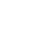) = I, where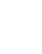is the optical depth of the cloud.

Let's connectto the physical parameters.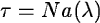N 		: 		 column density

a(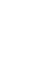:		 line absorption coefficient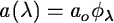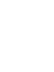ao =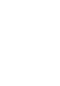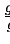l 		: 		lower level of the atomic transition

k 		: 		 upper level of the atomic transition: 		 rest wavelength of the transition

gl : 		 statistical weight of the lower level

gk : 		 statistical weight of the upper level

akl : 		 spontaneous transition probabilityflk = upward oscillator strength


Petra Nass
1999-06-15